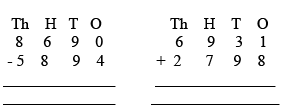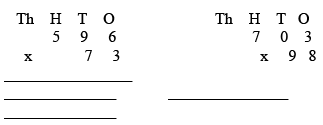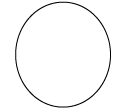Sample Test Paper: Mathematics

# Sample Test Paper: Mathematics Notes | Study How to Prepare for Admission Paper (Class 4) - Class 4

## Document Description: Sample Test Paper: Mathematics for Class 4 2022 is part of How to Prepare for Admission Paper (Class 4) for How to Prepare for Admission Paper (Class 4) preparation. The notes and questions for Sample Test Paper: Mathematics have been prepared according to the Class 4 exam syllabus. Information about Sample Test Paper: Mathematics covers topics like and Sample Test Paper: Mathematics Example, for Class 4 2022 Exam. Find important definitions, questions, notes, meanings, examples, exercises and tests below for Sample Test Paper: Mathematics.

Introduction of Sample Test Paper: Mathematics in English is available as part of our How to Prepare for Admission Paper (Class 4) for Class 4 & Sample Test Paper: Mathematics in Hindi for How to Prepare for Admission Paper (Class 4) course. Download more important topics related with How to Prepare for Admission Paper (Class 4), notes, lectures and mock test series for Class 4 Exam by signing up for free. Class 4: Sample Test Paper: Mathematics Notes | Study How to Prepare for Admission Paper (Class 4) - Class 4
 1 Crore+ students have signed up on EduRev. Have you?

A. Fill in the blanks:
1. Nine thousand three hundred and eighty six in figures is ___________.
2. The Place Value of  9 in  5 9 8 4 1 is ___________.
3. There are ___________ number of zeros in ten thousand.
4. The predecessor of 5000 is ___________.
5. The largest three digit number is ___________.
6. Half an hour is equal to ___________ minutes.
7. The product of  15 and 2 is ___________.
8. 1000 ml is equal to ___________ liters.
9. If the numerator is 4 and the denominator is 5 the fraction would be _________.
10. 96  - _________   = 80C.  Multiply:D. Find the quotient and remainder by dividing:
(i) 4934  by 8
(ii) 6792 by 5

E. Find the difference between 58000 and  24078.

F. Draw the hands of a clock to show 25 minutes past 11G. Add or subtract the fractions:
(i) 3/6 - 2/6 = ?
(ii) 7/9 + 5/9 = ?

H. Solve the following:
(i) There are 480 people on the train. If there are 12 compartments, how many people were in each compartment?
(ii) If there are 145 children in one class. How many children are there in 36 classes?

The document Sample Test Paper: Mathematics Notes | Study How to Prepare for Admission Paper (Class 4) - Class 4 is a part of the Class 4 Course How to Prepare for Admission Paper (Class 4).
All you need of Class 4 at this link: Class 4

## How to Prepare for Admission Paper (Class 4)

21 videos|3 docs
 Use Code STAYHOME200 and get INR 200 additional OFF

## How to Prepare for Admission Paper (Class 4)

21 videos|3 docs

Track your progress, build streaks, highlight & save important lessons and more!

,

,

,

,

,

,

,

,

,

,

,

,

,

,

,

,

,

,

,

,

,

;